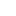# Unit 2: Supply and Demand

## Introduction

What’s in This Chapter? Why do prices of houses, cars, gasoline, and food fluctuate? What explains increases and decreases in interest rates? Why do prices of stocks and bonds change almost every second? Why does one gas...

## Section 1: The Law of Demand

Price and Quantity Changes The law of demand states that buyers of a good will purchase more of the good if its price is lower, and vice versa. This assumes that no other economic changes take place. If the price of apples...

## Section 2: The Demand Curve

Graphing the Demand Curve We can graph demand data in a diagram. The two variables we consider are the price of the product (P) and the amount of the product purchased during a certain period of time (Q). Economists measure the...

## Section 3: The Law of Supply

Price and Quantity Changes The law of supply states that, ceteris paribus, producers offer more of a product at higher than at lower prices. If the product price is high, the supplier can make greater profits by selling more...

## Section 4: The Supply Curve

Graphing the Supply Curve A supply curve slopes upward from the bottom left to the upper right of the diagram. At higher prices, firms are willing and able to sell more than at lower prices. We say that there is a direct...

## Section 5: Equilibrium Price and Quantity

The Market Price and Quantity In a free and competitive market without government price controls, the equilibrium, or market, price and quantity occur at the point at which the supply and demand curves intersect. At this price,...

## Section 6: Demand Determinants

Reasons for a Shift in the Demand Curve Demand can increase or decrease. In this case, the demand curve shifts to the right or to the left, respectively. The following are reasons: 1. A change in buyers’ real incomes or...

## Section 7: The Effect of a Change in Demand on Equilibrium Price and Quantity

An Increase in Demand Demand changes for any of the six reasons listed in the previous section. When the demand curve shifts to the right demand increases. The market price increases, as does the equilibrium quantity (in the...

## Section 8: Supply Determinants

Reasons for a Shift in the Supply Curve Supply can increase or decrease. In this case, the supply curve shifts to the right or to the left respectively. The following are reasons: 1. An advance in technology. An advance in the...

## Section 9: The Effect of a Change in Supply on Equilibrium Price and Quantity

An Increase in Supply Supply changes for any of the four reasons listed in the previous section. An increase in supply is illustrated by a rightward (or downward) shift of the supply curve. This decreases the price and increases...

## Section 10: The Effect of Changes in Both Demand and Supply on Equilibrium Price and Quantity

A Summary of how Demand and Supply Changes Affect Prices and Quantities The following summarizes the important relationships between changes in demand and supply and their corresponding equilibrium prices and equilibrium...

## Section 11: Demand versus Quantity Demanded and Supply versus Quantity Supplied

The Difference Between Demand and Quantity Demanded We learned in an earlier section that as the price of a product increases, the amount purchased by buyers decreases. This illustrates the law of demand. In a more recent...